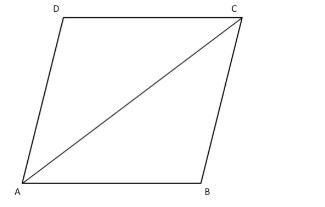# Find the area of a rhombus each side of which measures 20 cm and one of whose diagonals is 24 cm.`
Question:

Find the area of a rhombus each side of which measures 20 cm and one of whose diagonals is 24 cm.

Solution:

Given:
Sides are 20 cm each and one diagonal is of 24 cm.
The diagonal divides the rhombus into two congruent triangles, as shown in the figure below.We will now use Hero's formula to find the area of triangle ABC.
First, we will find the semiperimeter.

$s=\frac{1}{2}(a+b+c)=\frac{1}{2}(20+20+24)=\frac{64}{2}=32 \mathrm{~m}$

Area of $\Delta \mathrm{ABC}=\sqrt{s(s-a)(s-b)(s-c)}$ $=\sqrt{32(32-20)(32-20)(32-24)}$

$=\sqrt{32 \times 12 \times 12 \times 8}$

$=\sqrt{36864}$

$=192 \mathrm{~cm}^{2}$

Now,

Area of the rhombus $=2 \times$ Area of triangle $\mathrm{ABC}=192 \times 2=384 \mathrm{~cm}^{2}$